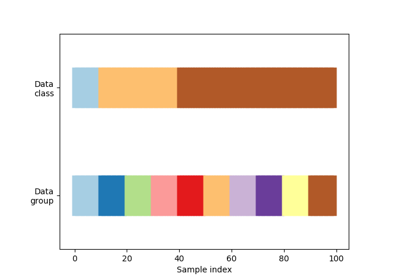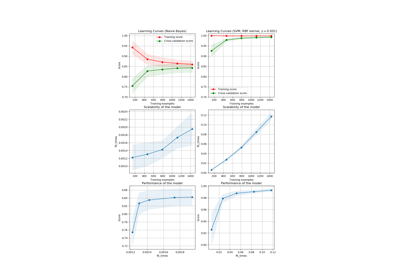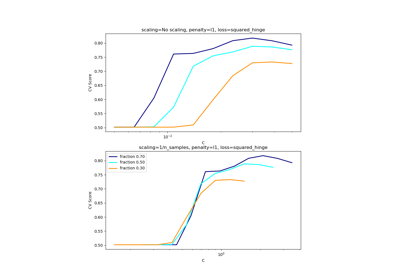# sklearn.model_selection.ShuffleSplit¶

class sklearn.model_selection.ShuffleSplit(n_splits=10, test_size=None, train_size=None, random_state=None)[source]

Random permutation cross-validator

Yields indices to split data into training and test sets.

Note: contrary to other cross-validation strategies, random splits do not guarantee that all folds will be different, although this is still very likely for sizeable datasets.

Read more in the User Guide.

Parameters
n_splitsint, default 10

Number of re-shuffling & splitting iterations.

test_sizefloat, int, None, default=None

If float, should be between 0.0 and 1.0 and represent the proportion of the dataset to include in the test split. If int, represents the absolute number of test samples. If None, the value is set to the complement of the train size. If train_size is also None, it will be set to 0.1.

train_sizefloat, int, or None, default=None

If float, should be between 0.0 and 1.0 and represent the proportion of the dataset to include in the train split. If int, represents the absolute number of train samples. If None, the value is automatically set to the complement of the test size.

random_stateint, RandomState instance or None, optional (default=None)

If int, random_state is the seed used by the random number generator; If RandomState instance, random_state is the random number generator; If None, the random number generator is the RandomState instance used by np.random.

Examples

>>> import numpy as np
>>> from sklearn.model_selection import ShuffleSplit
>>> X = np.array([[1, 2], [3, 4], [5, 6], [7, 8], [3, 4], [5, 6]])
>>> y = np.array([1, 2, 1, 2, 1, 2])
>>> rs = ShuffleSplit(n_splits=5, test_size=.25, random_state=0)
>>> rs.get_n_splits(X)
5
>>> print(rs)
ShuffleSplit(n_splits=5, random_state=0, test_size=0.25, train_size=None)
>>> for train_index, test_index in rs.split(X):
...     print("TRAIN:", train_index, "TEST:", test_index)
TRAIN: [1 3 0 4] TEST: [5 2]
TRAIN: [4 0 2 5] TEST: [1 3]
TRAIN: [1 2 4 0] TEST: [3 5]
TRAIN: [3 4 1 0] TEST: [5 2]
TRAIN: [3 5 1 0] TEST: [2 4]
>>> rs = ShuffleSplit(n_splits=5, train_size=0.5, test_size=.25,
...                   random_state=0)
>>> for train_index, test_index in rs.split(X):
...     print("TRAIN:", train_index, "TEST:", test_index)
TRAIN: [1 3 0] TEST: [5 2]
TRAIN: [4 0 2] TEST: [1 3]
TRAIN: [1 2 4] TEST: [3 5]
TRAIN: [3 4 1] TEST: [5 2]
TRAIN: [3 5 1] TEST: [2 4]


Methods

 get_n_splits(self[, X, y, groups]) Returns the number of splitting iterations in the cross-validator split(self, X[, y, groups]) Generate indices to split data into training and test set.
__init__(self, n_splits=10, test_size=None, train_size=None, random_state=None)[source]

Initialize self. See help(type(self)) for accurate signature.

get_n_splits(self, X=None, y=None, groups=None)[source]

Returns the number of splitting iterations in the cross-validator

Parameters
Xobject

Always ignored, exists for compatibility.

yobject

Always ignored, exists for compatibility.

groupsobject

Always ignored, exists for compatibility.

Returns
n_splitsint

Returns the number of splitting iterations in the cross-validator.

split(self, X, y=None, groups=None)[source]

Generate indices to split data into training and test set.

Parameters
Xarray-like, shape (n_samples, n_features)

Training data, where n_samples is the number of samples and n_features is the number of features.

yarray-like, shape (n_samples,)

The target variable for supervised learning problems.

groupsarray-like, with shape (n_samples,), optional

Group labels for the samples used while splitting the dataset into train/test set.

Yields
trainndarray

The training set indices for that split.

testndarray

The testing set indices for that split.

Notes

Randomized CV splitters may return different results for each call of split. You can make the results identical by setting random_state to an integer.

## Examples using sklearn.model_selection.ShuffleSplit¶